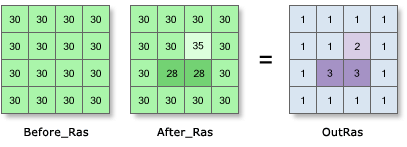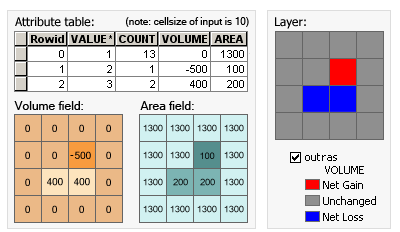Cut Fill (Spatial Analyst)

Summary

Calculates the volume change between two surfaces. This is typically used for cut and fill operations.

IllustrationOutRas = CutFill(Before_Ras, After_Ras)When the Cut Fill operation is performed, by default, a specialized renderer is applied to the layer that highlights the locations of cut and of fill. The determinant is in the attribute table of the output raster, which considers positive volume to be where material was cut (removed), and negative volume where material was filled (added).

Usage

• The Cut Fill tool enables you to create a map based on two input surfaces—before and after—displaying the areas and volumes of surface materials that have been modified by the removal or addition of surface material.

• Both the input raster surfaces must be coincident. That is, they must have a common origin, the same number of rows and columns of cells, and the same cell size.

• For accurate results, the z-units should be the same as the x,y ground units. This ensures that the resulting volumes are meaningful cubic measures (for example, cubic meters). If they are not the same, use a z-factor to convert z units to x,y units. For example, if your x,y units are meters and your z units are feet, you could specify a z-factor of 0.3048 to convert feet to meters.

Alternatively, use the Times math tool to create a surface raster in which the z-values have been adjusted to correspond to the ground units.

• The attribute table of the output raster presents the changes in the surface volumes following the cut/fill operation. Positive values for the volume difference indicate regions of the before raster surface that have been cut (material removed). Negative values indicate areas that have been filled (material added). See How Cut Fill works for more details on how the results are calculated.

• When the cut/fill operation is performed from the tool, by default a specialized renderer is applied that highlights the locations of cut and of fill. The renderer draws areas that have been cut in blue, and areas that have been filled in red. Areas that have not changed are displayed in grey.

• When the input raster needs to be resampled, the bilinear technique will be used. An example of when an input raster may be resampled is when the output coordinate system, extent, or cell size is different from that of the input.

• See Analysis environments and Spatial Analyst for additional details on the geoprocessing environments that apply to this tool.

Syntax

CutFill(in_before_surface, in_after_surface, {z_factor})
 Parameter Explanation Data Type in_before_surface The input representing the surface before the cut or fill operation. Raster Layer in_after_surface The input representing the surface after the cut or fill operation. Raster Layer z_factor(Optional) Number of ground x,y units in one surface z-unit.The z-factor adjusts the units of measure for the z-units when they are different from the x,y units of the input surface. The z-values of the input surface are multiplied by the z-factor when calculating the final output surface.If the x,y units and z-units are in the same units of measure, the z-factor is 1. This is the default.If the x,y units and z-units are in different units of measure, the z-factor must be set to the appropriate factor, or the results will be incorrect. For example, if your z-units are feet and your x,y units are meters, you would use a z-factor of 0.3048 to convert your z-units from feet to meters (1 foot = 0.3048 meter). Double

Return Value

 Name Explanation Data Type out_raster The output raster defining regions of cut and of fill.The values show the locations and amounts where the surface has been added to or removed from. Raster

Code sample

CutFill example 1 (Python window)

This example calculates the volume and area of cut and fill locations and outputs the result as a Grid raster.

import arcpy
from arcpy import env
from arcpy.sa import *
env.workspace = "C:/sapyexamples/data"
outCutFill = CutFill("elevation01", "elevation02", 1)
outCutFill.save("C:/sapyexamples/output/outcutfill01")
CutFill example 2 (stand-alone script)

This example calculates the volume and area of cut and fill locations and outputs the result as a Grid raster.

# Name: Cutfill_Ex_02.py
# Description: Calculates the volume and area of cut and
#              fill locations.
# Requirements: Spatial Analyst Extension

# Import system modules
import arcpy
from arcpy import env
from arcpy.sa import *

# Set environment settings
env.workspace = "C:/sapyexamples/data"

# Set local variables
inBeforeRaster = "elevation01"
inAfterRaster =  "elevation02"
zFactor = 0.5

# Execute CutFill
outCutFill = CutFill(inBeforeRaster, inAfterRaster, zFactor)

# Save the output
outCutFill.save("C:/sapyexamples/output/outcutfill02")

Licensing information

• Basic: Requires Spatial Analyst or 3D Analyst
• Standard: Requires Spatial Analyst or 3D Analyst
• Advanced: Requires Spatial Analyst or 3D Analyst